# Divisibility Tests 2-12

## Divisibility Tests:

A number is divisible by 2 if its last digit is even (0,2,4,6 or 8)

A number is divisible by 3 if the sum of its digits is divisible by 3.

A number is divisible by 4 if the number's last two digits are divisible by 4.

A number is divisible by 5 if its last digit is a 0 or 5.

A number is divisible by 6 if it is divisible by 2 and 3 (see rules above).

A number is divisible by 7 if 5 times the last digit added to the number made from the other digits is divisible by 7.

A number is divisible by 8 if the last three digits form a number that is divisible 8.

A number is divisible by 9 if the sum of the digits is divisible by 9.

A number is divisible by 10 if its last digit is 0.

A number is divisible by 11 if the alternating sum of its digits is divisible by 11. Alternating sum means a-b+c-d+... – m

A number is divisible by 12 if it is divisible by 3 and 4.

The projectable version of the divisibility tests can be found here: Divisibility Tests 2-12

There is a printable worksheet to go with this activity.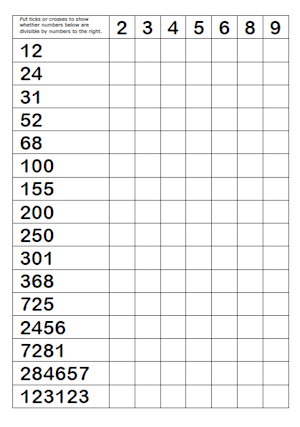The divisibility test for 7 is thanks to a 12-year old pupil, Chika Ofili, from Westminster School. You can read more about it here.

#### Divisibility Test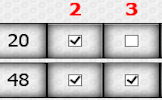Practise using the quick ways to spot whether a number is divisible by the digits two to nine.

Transum.org/go/?to=divisibility

#### Divides Exactly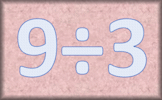Find which divisions result in whole number quotients. A mental arithmetic speed challenge.

Transum.org/go/?to=dividesexactly

#### Divisibility Tests Worksheet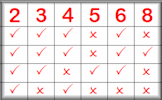This worksheet contains a list of the divisibility tests along with a fill-in-the-table exercise.

Transum.org/Maths/Worksheet/?name=divtestprint

#### Delightfully Divisible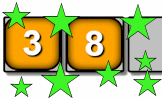Arrange the digits one to nine to make a number which is divisible in the way described.

Transum.org/go/?to=delight

#### Jugs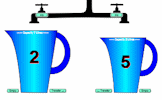The classic problem of using two unmarked jugs to measure exactly a given quantity. The Transum version is interactive and awards a virtual trophy for each of the levels completed.

This is a Shine+Write visual aid designed to be projected onto a whiteboard for whole class exposition.

You can find other popular visual aids on our Shine+Write page.

Find out about the latests developments on this website by signing up for a Transum Subscription. You will receive a regular newsletter and have access to the answers to most of the puzzles and activities on this site.

Transum,

Sunday, September 22, 2019

"The divisibility test for 7 is thanks to a 12-year old pupil, Chika Ofili, from Westminster School. You can read more about him here.

In a bored moment, Chika had turned his mind to finding a divisibility test for seven and this is what he came up with. He realised that if you take the last digit of any whole number, multiply it by 5 and then add this to the remaining part of the number, you will get a new number. And it turns out that if this new number is divisible by 7, then the original number is divisible by 7. What an easy test!

For example, take the number 532
53 + 2 x 5 = 63
63 is a multiple of 7, so 532 is divisible by 7

Well done Chika!"

Do you have any comments? It is always useful to receive feedback and helps make this free resource even more useful for those learning Mathematics anywhere in the world. Click here to enter your comments.

#### Hexagram StarArrange the twelve numbers on the hexagram so that the numbers in each line add up to the same total. So far this activity has been accessed 156 times and 5 people have earned a Transum Trophy for completing it.For All: